• 实验三 最小错误率贝叶斯分类 利用贝叶斯后验概率确定分类：设有19人进行体检，结果如下表。但事后发现4人忘了写性别，试问，这4人是男是女？ 代码 function self_mvnrnd1(varargin)%可自定义参数的函数 if...

实验三 最小错误率的贝叶斯分类

利用贝叶斯后验概率确定分类：设有19人进行体检，结果如下表。但事后发现4人忘了写性别，试问，这4人是男是女？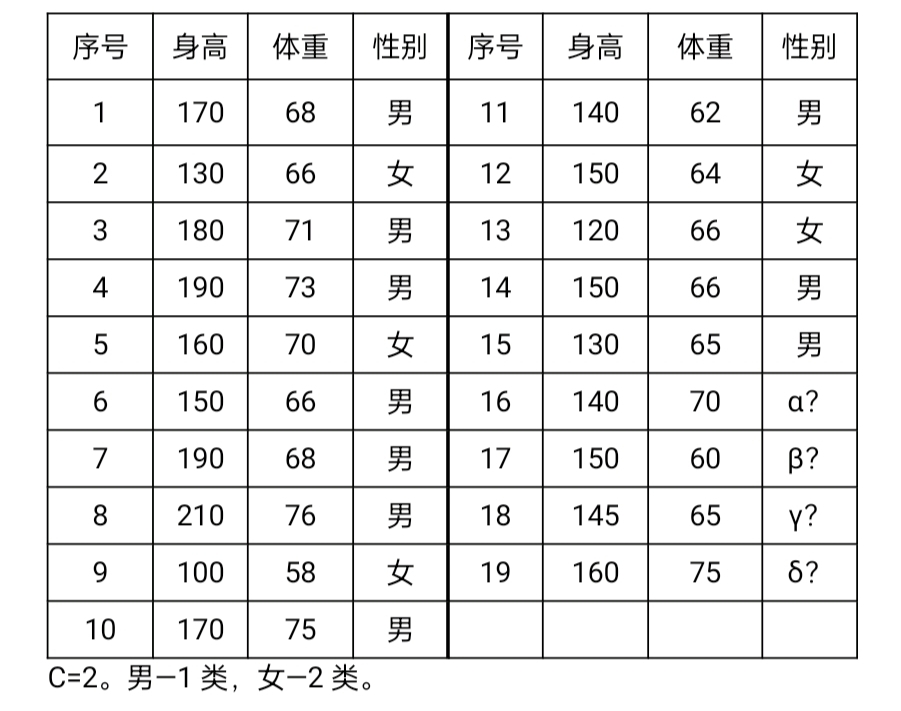代码

import xlrd
import math
import numpy as np
import scipy.stats as st
import matplotlib.pyplot as plt
from scipy.stats import norm

‘’’
Take the height as an example, draw a histogram of the
height of the boys and girls and compare
‘’’
mydata = xlrd.open_workbook(‘D:/program/py_code/data_2018.xls’)
mysheet1 = mydata.sheet_by_name(“Sheet1”)

#获取行数、列数
nRows=mysheet1.nrows
nCols=mysheet1.ncols

#用于存取男生女生身高数据
man_height=[]
woman_height=[]

#获取第4列的内容:身高
for i in range(nRows):
if i+1<nRows:
if mysheet1.cell(i+1,1).value1:
man_height.append(mysheet1.cell(i+1,3).value)
elif mysheet1.cell(i+1,1).value
0:
woman_height.append(mysheet1.cell(i+1,3).value)

#获取男、女生的数量
manlen=len(man_height)
womanlen=len(woman_height)

#画男女生身高频谱图
plt.hist(man_height,manlen,align=‘left’,color=‘red’,label=‘boy’)
plt.hist(woman_height,womanlen,align=‘right’,label=‘girl’)
plt.legend(loc=0)
plt.xlabel(‘height’)
plt.xlim(min(man_height+woman_height)-1,max(man_height+woman_height)+1)
plt.ylabel(‘number’)
plt.title(‘Boy height spectrum’)
#xsticks与yticks：指定坐标轴的刻度
plt.xticks(np.arange(min(man_height+woman_height),max(man_height+woman_height)+1,1.0))
plt.yticks(np.linspace(0,50,26))
plt.show()

‘’’
Using the maximum likelihood estimation method to find the
parameters of height and weight distribution for boys and girls
‘’’
#用于存取男生女生体重数据
man_weight=[]
woman_weight=[]

#将男女生体重数据从第5列中进行分离，并保存在上述空数组中
for i in range(nRows):
if i+1<nRows:
if mysheet1.cell(i+1,1).value1:
man_weight.append(mysheet1.cell(i+1,4).value)
elif mysheet1.cell(i+1,1).value
0:
woman_weight.append(mysheet1.cell(i+1,4).value)
#fit(data):Return MLEs for shape, location, and scale parameters from data
#norm.fit(x)就是将x看成是某个norm分布的抽样，求出其最好的拟合参数（mean, std）
man_height_mean, man_height_std = norm.fit(man_height)#男生升高分布参数
man_weight_mean, man_weight_std = norm.fit(man_weight)#男生体重分布参数
woman_height_mean, woman_height_std = norm.fit(woman_height)#女生升高分布参数
woman_weight_mean, woman_weight_std = norm.fit(woman_weight)#女生体重分布参数

man_height_variance=man_height_std2
man_weight_variance=man_weight_std
2
woman_height_variance=woman_height_std2
woman_weight_variance=woman_weight_std
2
print(man_height_mean,man_height_variance,man_weight_mean,man_weight_variance)
print(woman_height_mean,woman_height_variance,woman_weight_mean,woman_weight_variance)

‘’’
采用贝叶斯估计方法，求男女生身高以及体重分布的参数（注明自己选定的参数情况）
‘’’
man_height_mean_cntr, man_height_var_cntr, man_height_std_cntr=st.bayes_mvs(man_height)
man_weight_mean_cntr, man_weight_var_cntr, man_weight_std_cntr=st.bayes_mvs(man_weight)
woman_height_mean_cntr, woman_height_var_cntr, woman_height_std_cntr=st.bayes_mvs(woman_height)
woman_weight_mean_cntr, woman_weight_var_cntr, woman_weight_std_cntr=st.bayes_mvs(woman_weight)
#print(man_height_mean_cntr.statistic,man_weight_mean_cntr.statistic)
#print(woman_height_mean_cntr.statistic,woman_weight_mean_cntr.statistic)

def get_mean_bayes(arr,mean0,variance0,variance):
datasum=sum(arr)
datalen=len(arr)
mean_bayes=(variance0datasum+variancemean0)/(datalen*variance0+variance)
return mean_bayes

‘’’
特殊情况1:
variance0=0时，mean_bayes=mean0
先验知识可靠，样本不起作用
#以男生身高为例，选定参数：mean0=20,variance=10,variance0=0
‘’’
man_height_mean_bayes=get_mean_bayes(man_height,20,0,10)
print(man_height_mean_bayes)

‘’’
特殊情况2:
variance0>>variance时，mean_bayes=sample_mean
先验知识十分不确定，完全依靠样本信息,结果与最大似然估计结果近似
#以女生身高为例，选定参数：mean0=50,variance=1,variance0=100
‘’’
woman_height_mean_bayes=get_mean_bayes(woman_height,50,100,1)
print(woman_height_mean_bayes)

‘’’
4. 采用最小错误率贝叶斯决策，画出类别判定的决策面。并判断某样本
的身高体重分别为(160,45)时应该属于男生还是女生？为(178,70)时呢？
‘’’
#①本题输入类数为2：即男生、女生；特征数为2：即身高、体重
#②求协方差矩阵
def get_covariance_matrix_coefficient(arr1,arr2):#arr1与arr2长度相等
datalength1=len(arr1)
datalength2=len(arr2)
sum_temp=[]
for i in range(datalength1):
sum_temp.append((arr1[i]-sum(arr1)/datalength1)*(arr2[i]-sum(arr2)/datalength2))
c12=sum(sum_temp)
covariance_matrix_c12=c12/(datalength1-1)
return covariance_matrix_c12

man_c11=man_height_variance
man_c22=man_weight_variance
man_c12=man_c21=get_covariance_matrix_coefficient(man_height,man_weight)
man_covariance_matrix=np.matrix([[man_c11,man_c12],[man_c21,man_c22]])
woman_c11=woman_height_variance
woman_c22=woman_weight_variance
woman_c12=woman_c21=get_covariance_matrix_coefficient(woman_height,woman_weight)
woman_covariance_matrix=np.matrix([[woman_c11,woman_c12],[woman_c21,woman_c22]])
print(woman_covariance_matrix)

#求男生、女生先验概率
man_priori_probability=manlen/(manlen+womanlen)
woman_priori_probability=1-man_priori_probability
#print(woman_priori_probability)

man_feature_mean_vector=np.matrix([[man_height_mean],[man_weight_mean]])
woman_feature_mean_vector=np.matrix([[woman_height_mean],[woman_weight_mean]])

定义等高线高度函数

def f(sample_height, sample_weight):
mytemp1=np.zeros(shape=(100,100))
for i in range(100):
for j in range(100):
sample_vector=np.matrix([[sample_height[i,j]],[sample_weight[i,j]]])
sample_vector_T=np.transpose(sample_vector)
#定义决策函数
mytemp1[i,j]=0.5np.transpose(sample_vector-man_feature_mean_vector)(np.linalg.inv(man_covariance_matrix))
(sample_vector-man_feature_mean_vector)-0.5
np.transpose(sample_vector-woman_feature_mean_vector)
(np.linalg.inv(woman_covariance_matrix))
(sample_vector-woman_feature_mean_vector)+
0.5*math.log((np.linalg.det(man_covariance_matrix))/(np.linalg.det(woman_covariance_matrix)))-
math.log(man_priori_probability/woman_priori_probability)
return mytemp1

sample_height = np.linspace(150, 180, 100)
sample_weight = np.linspace(40, 80, 100)

将原始数据变成网格数据

Sample_height, Sample_weight = np.meshgrid(sample_height, sample_weight)

填充颜色

plt.contourf(Sample_height, Sample_weight, f(Sample_height,Sample_weight), 0, alpha = 0)

绘制等高线,圈内为女生，圈外为男生

C = plt.contour(Sample_height, Sample_weight, f(Sample_height,Sample_weight), 0, colors = ‘black’,linewidths=0.6)

显示各等高线的数据标签

plt.clabel(C, inline = True, fontsize = 10)

#显示男女生样本散点图

p1=plt.scatter(man_height, man_weight,c=‘g’, marker = ‘’,linewidths=0.4)
p2=plt.scatter(woman_height, woman_weight,c=‘r’, marker = '
’,linewidths=0.4)

定义显示坐标函数

def Display_coordinates(m, n):
plt.scatter(m, n, marker = ‘s’,linewidths=0.4)
plt.annotate((m,n), xy = (m, n))
return

#并判断某样本的身高体重分别为(160,45)时应该属于男生还是女生？为(178,70)时呢
Display_coordinates(160,45)
Display_coordinates(178,70)
label=[‘boy’,‘girl’]
plt.legend([p1, p2],label,loc=0)
plt.xlabel(‘height/cm’)
plt.ylabel(‘weight/kg’)
plt.show()
实验结果展开全文r语言 机器学习 sklearn
• 假定某个局部区域细胞识别中正常P(w1)和异常P（w2)两类先验概率分别为P(w1)=0.9, P(w2)=0.1现有一系列待观察的细胞，其观察值为：-2.67 -3.55 -1.24 -0.98 -0.79 -...依据最小错误率贝叶斯决策对观察的结果进行分类
• 最小错误率贝叶斯分类器（包含matlab程序）1. 最小错误贝叶斯分类器原理2.最小错误率贝叶斯分类器的图形表示3.三类最小错误率贝叶斯分类器实验实验代码 1. 最小错误贝叶斯分类器原理 在对模式进行识别时，在存在...

1. 最小错误贝叶斯分类器原理

在对模式进行识别时，在存在模棱两可的情况下，任何决策都存在判别错误的可能性。最小错误贝叶斯决策就是以错误率为自小的分类规则。使用p(e)表示错误概率，那么最小错误贝叶斯分类器的目的就是minp(e).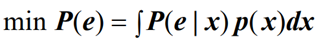对于两种模式，其中：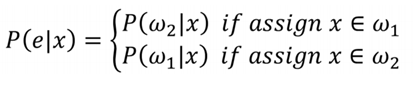即x属于第一类，判别为第二类的概率，x属于第二类，判别为第一类的概率。
可以看出使错误率最小的决策就是使后验概率最大的决策，因此最小错误率贝叶斯决策可写为：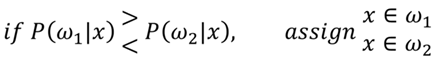其中，后验概率的计算使用贝叶斯公式计算，因此要已知先验概率p(ωi)以及类条件概率密度p(x|wi)。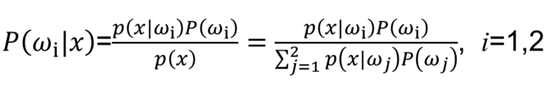判决公式还有很多等价公式。

2.最小错误率贝叶斯分类器的图形表示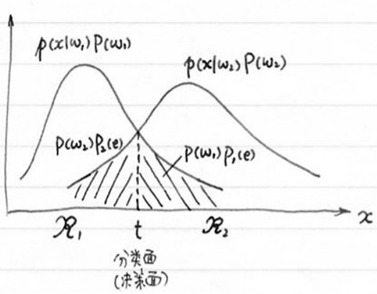错误率：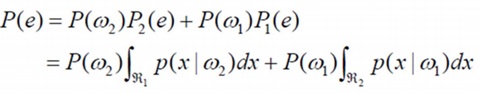对于多类问题的决策，思路跟两类问题类似，不同的只是计算量加重了许多；可以把每一类的后验概率当做是该类的一个判别函数gi(x),决策的过程就是各类的判别函数进行比较，最后取最大的那个。使用图形表示为：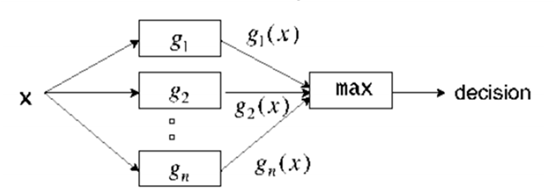3.三类最小错误率贝叶斯分类器实验

（1）使用函数产生mvnrnd三类服从高斯分布的样本点。高斯函数的参数作为输入参数输入，包括均值，协方差，个数，先验概率。
（2）为了区分三类样本，第一类使用蓝色，第二类使用绿色，第三类使用红色。
第一类错分的点使用黑色三角表示下来，第二类错分的店使用黄色+表示下来，第三类使用粉红色正方形表示出来。
（3）使用函数mvnpdf()实现高斯密度函数值的计算
（4）比较高斯密度值的大小，选取最大的一类，分到那一类。

输入参数分别为：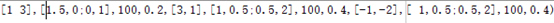实验结果：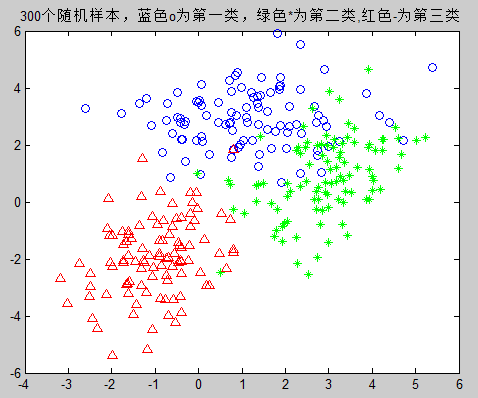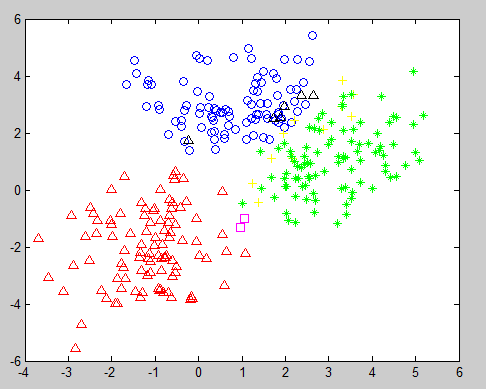实验代码

function self_mvnrnd1(varargin)%可自定义参数的函数
if(nargin==12)%判定输入参数是否为12
w1=mvnrnd(varargin{1},varargin{2},varargin{3});%第一类高斯函数密度值
w2=mvnrnd(varargin{5},varargin{6},varargin{7});%第二类
w3=mvnrnd(varargin{9},varargin{10},varargin{11});
figure(1);
plot(w1(:,1),w1(:,2),'bo');%蓝色o为第一类
hold on
plot(w2(:,1),w2(:,2),'g*');%绿色*为第二类
hold on
plot(w3(:,1),w3(:,2),'r^')
title('300个随机样本，蓝色o为第一类，绿色*为第二类,红色-为第三类');
w=[w1;w2;w3];
n1=0;%第一类正确个数
n2=0;%第二类正确个数
n3=0;%第三类正确的个数
figure(2);
%贝叶斯分类器
for i=1:(varargin{3}+varargin{7}+varargin{11})
x=w(i,1);
y=w(i,2);
g1=mvnpdf([x,y],varargin{1},varargin{2})*varargin{4};
g2=mvnpdf([x,y],varargin{5},varargin{6})*varargin{8};
g3=mvnpdf([x,y],varargin{9},varargin{10})*varargin{12};
if g1>g3&&g1>g2
if 1<=i&&i<=varargin{3}
n1=n1+1;%第一类正确个数
plot(x,y,'bo');%蓝色o表示正确分为第一类的样本
hold on;
else
plot(x,y,'k^');%红色的上三角形表示第一类错误分为第二类
hold on;
end
end
if g2>g3&&g2>g1
if varargin{3}<=i&&i<=(varargin{3}+varargin{7})
n2=n2+1;%第一类正确个数
plot(x,y,'g*');%绿色o表示正确分为第二类的样本
hold on;
else
plot(x,y,'y+');%红色的上三角形表示第一类错误分为第二类
hold on;
end
end
if g3>g1&&g3>g2
if (varargin{3}+varargin{7})<=i&&i<=(+varargin{7}+varargin{3}+varargin{11})
n3=n3+1;%第一类正确个数
plot(x,y,'r^');%绿色o表示正确分为第二类的样本
hold on;
else
plot(x,y,'ms');%红色的上三角形表示第一类错误分为第二类
hold on;
end
end
end
r1_rate=0;
r2_rate=0;
r3_rate=0;
r1_rate=n1/varargin{3}
r2_rate=n2/varargin{7}
r3_rate=n3/varargin{11}
print(r1_rate)
print(r2_rate)
print(r3_rate)
end
%%%%%%%%%
main.m
self_mvnrnd1([1 3],[1.5,0;0,1],100,0.2,[3,1],[1,0.5;0.5,2],100,0.4,[-1,-2],[ 1,0.5;0.5,2],100,0.4)

/

展开全文分类器 matlab
• 基于最小错误率贝叶斯手写数字分类器，包括注释，很详尽，是自己按照定义写的，训练集和测试集是用的mnist数据集，最终正确率为73.89%
• 最小错误率贝叶斯分类器

万次阅读 2017-03-29 19:32:14
本次实验的主要内容是编程实现一个可以对两类模式样本进行分类的贝叶斯分类器，其中假设两个模式类的条件概率分布均为高斯分布。本次实验自定义一个函数self_mvnrnd(varargin) ，输入8个参数，其中，参数1，2，3，4...

一、 技术论述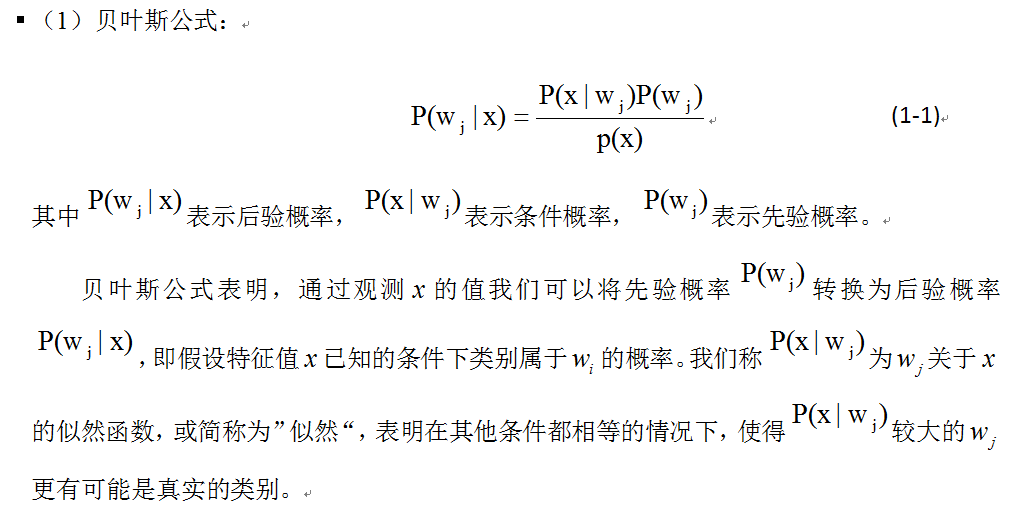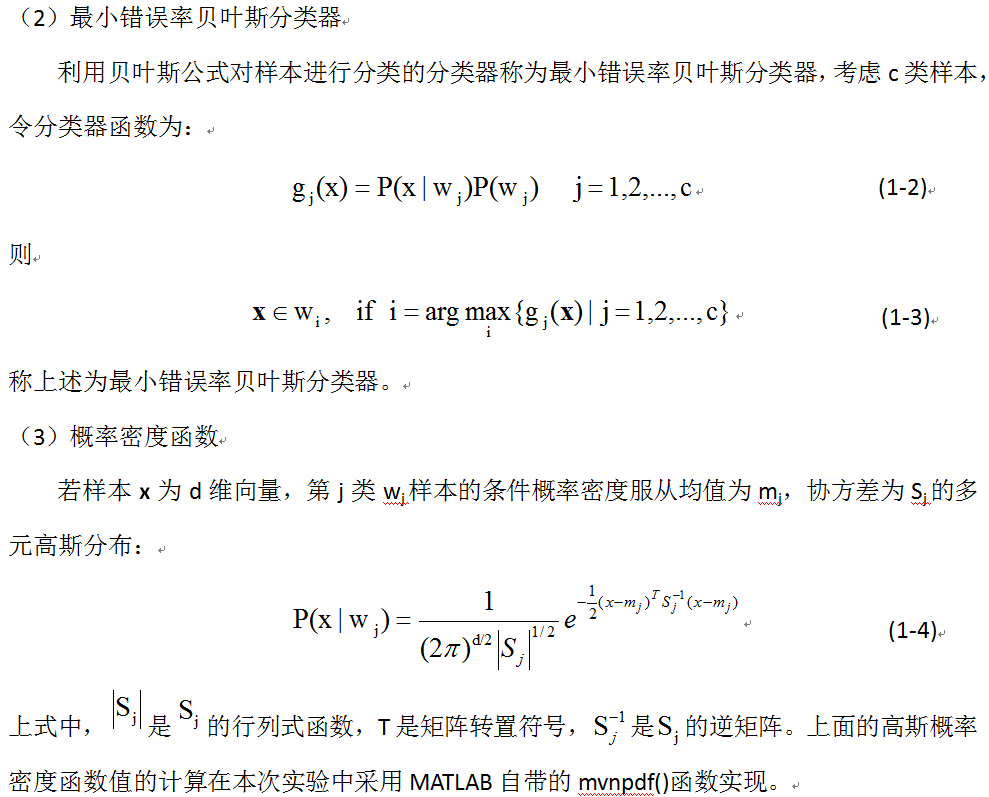二、 实验步骤及实验结果

1、基本实验

(1) 生成两类模式样本，模式类1的均值矢量为m1=(1,3)T，协方差矩阵为S1=(1.5,0;0,1)，模式类2的均值矢量为m2=(3,1)T，协方差矩阵为S2=(1,0.5;0.5,2)，先验概率P(w1)=P(w2)=0.5。在MATLAB中为两个模式类各生成100个随机样本点，并在一幅图中分别用蓝色和绿色画出这两类样本的二维散点图，其中红色的上三角形表示第一类错误分为第二类，红色的下三角形表示第二类错误分为第一类，实验结果如图1所示：
具体调用函数：self_mvnrnd([1 3],[1.5,0;0,1],100,0.5,[3,1],[1,0.5;0.5,2],100,0.5)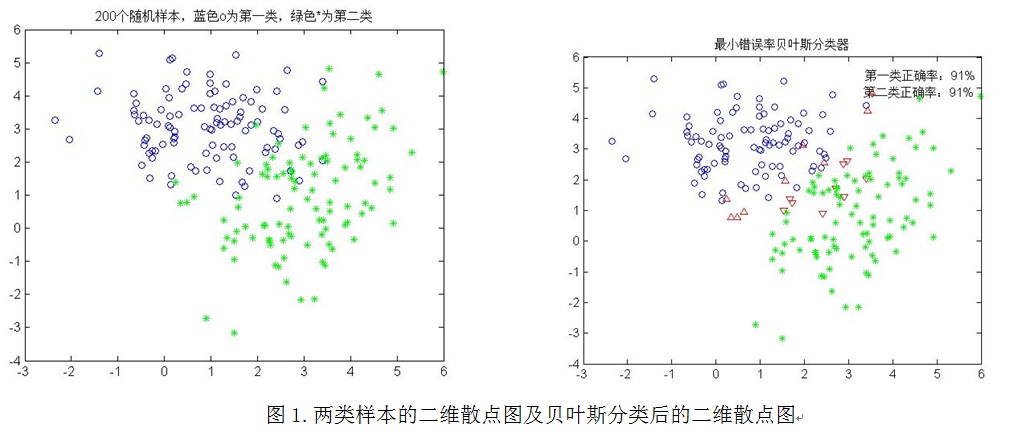从图1分类后的二维散点图可知正确分类的百分比：第一类为91%，第二类为91%。

(2) 改变先验概率P(w1)=0.4，P(w2)=0.6，对上述200个样本重新进行分类，实验结果如下图2所示：
改变先验概率，具体调用函数：self_mvnrnd([1 3],[1.5,0;0,1],100,0.4,[3,1],[1,0.5;0.5,2],100,0.6)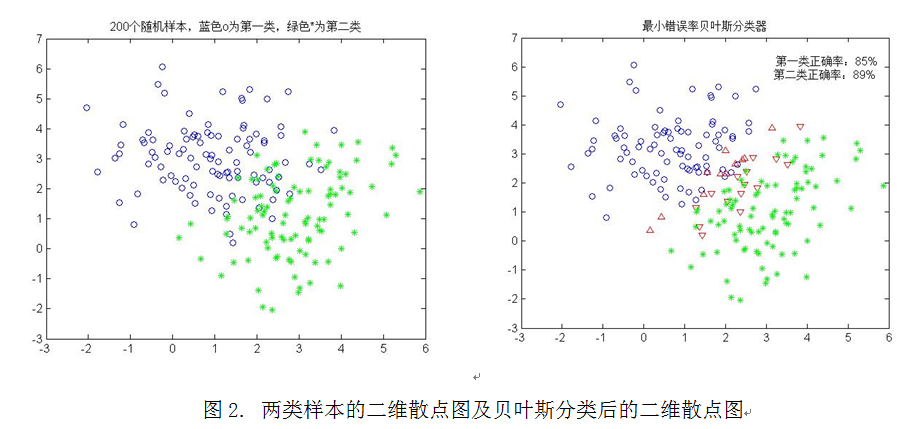从图2可以看出，正确分类的百分比：第一类为85%，第二类为89%。

2、 扩展实验

(1) 在上述基本实验中，协方差矩阵不变，但类均值向量分别变为m1=(1,3)T，m2=(2,2)T，重新进行基本实验中(1)、(2)、(3)的实验，得到实验结果如下图3所示：
具体调用函数：self_mvnrnd([1 3],[1.5,0;0,1],100,0.5,[2,2],[1,0.5;0.5,2],100,0.5)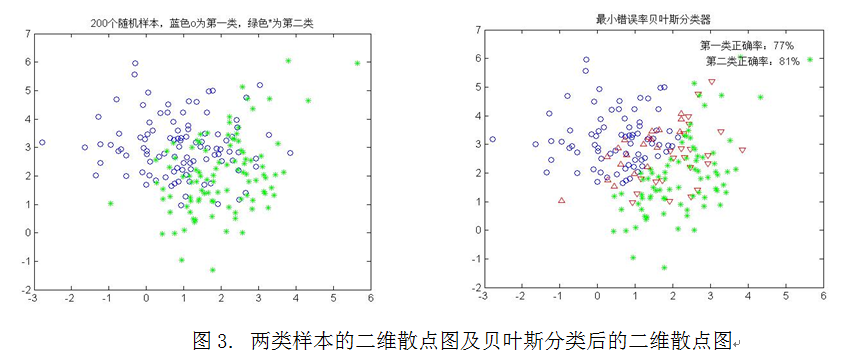从图3可以看出正确分类的百分比：第一类为77%，第二类为81%。

改变先验概率，具体调用函数：self_mvnrnd([1 3],[1.5,0;0,1],100,0.4,[2,2],[1,0.5;0.5,2],100,0.6)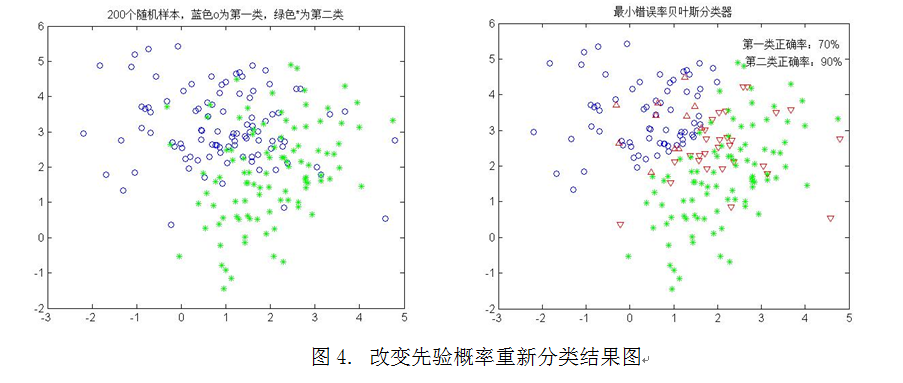从图4可以看出正确分类的百分比：第一类为70%，第二类为90%。

(2) 在1实验中，协方差矩阵不变，但类均值向量分别变为m1=(1,3)T，m2=(4,0)T，重新进行基本实验中(1)、(2)、(3)的实验，得到实验结果如下图5所示：
具体调用函数：self_mvnrnd([1 3],[1.5,0;0,1],100,0.5,[4,0],[1,0.5;0.5,2],100,0.5)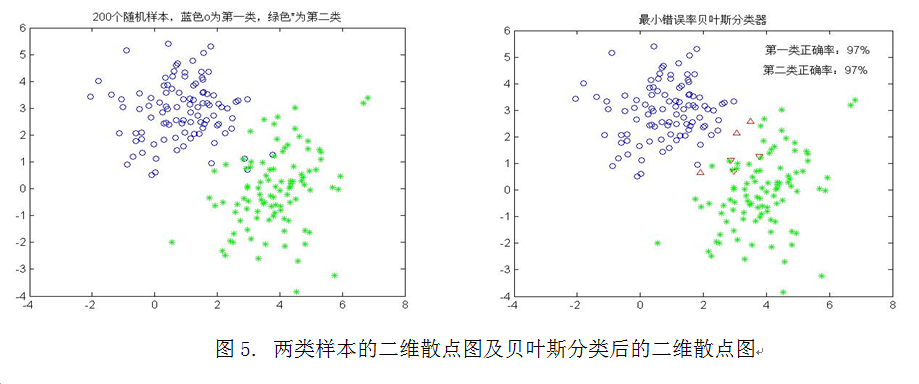从图5可以看出正确分类的百分比：第一类为97%，第二类为97%。

改变先验概率，具体调用函数：self_mvnrnd([1 3],[1.5,0;0,1],100,0.4,[4,0],[1,0.5;0.5,2],100,0.6)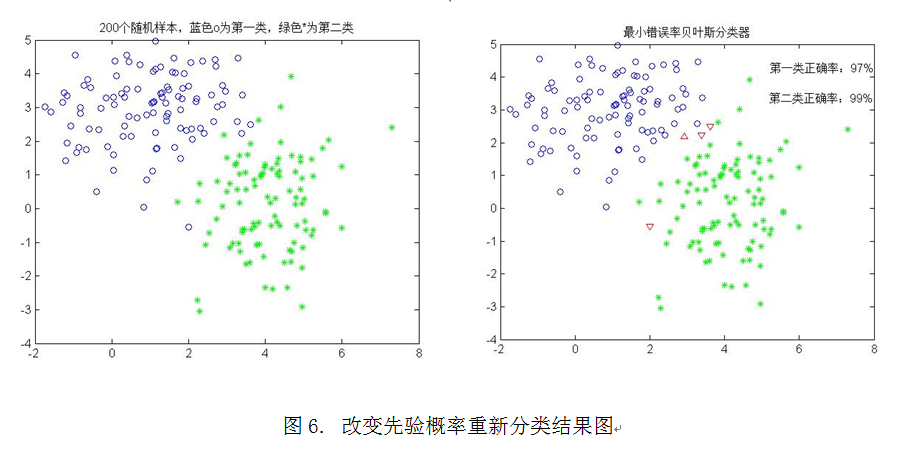从图6可以看出正确分类的百分比：第一类为97%，第二类为99%。

(3)在1实验中，两个类均值向量不变，但协方差矩阵分别变为S1=(1.5,1;1,1)，S2=(1,0.5;0.5,2)重新进行基本实验中(1)、(2)、(3)的实验，实验结果如下图7所示：
具体调用函数：self_mvnrnd([1 3],[1.5,1;1,1],100,0.5,[3,1],[1,0.5;0.5,2],100,0.5)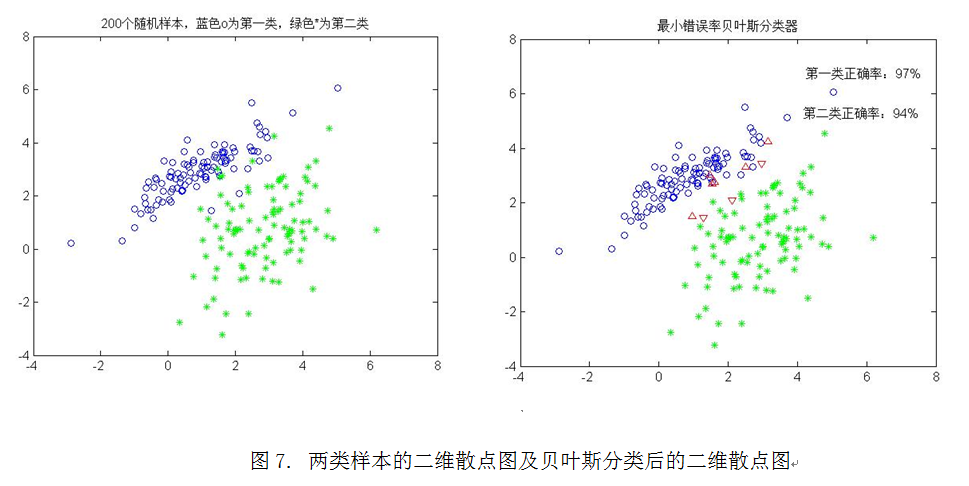从图7可以看出正确分类的百分比：第一类为97%，第二类为94%。

改变先验概率，具体调用函数：self_mvnrnd([1 3],[1.5,1;1,1],100,0.4,[3,1],[1,0.5;0.5,2],100,0.6)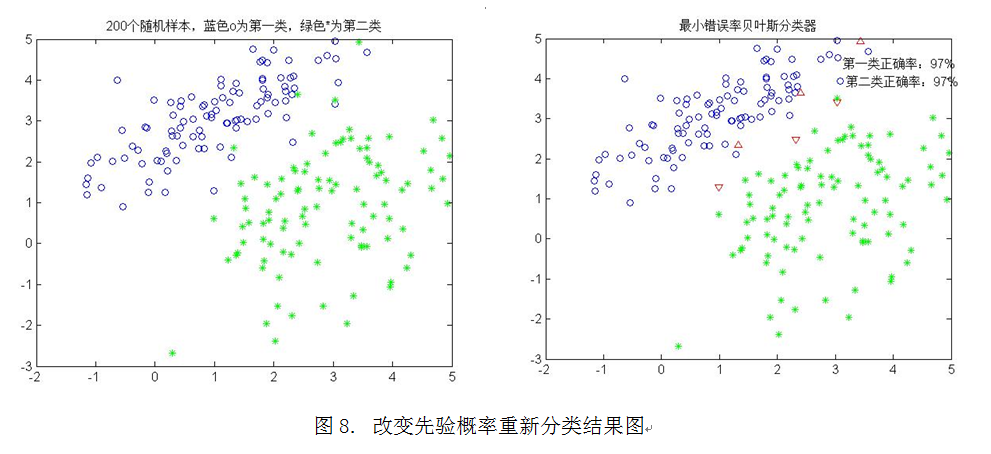从图8可以看出正确分类的百分比：第一类为97%，第二类为97%。

三、 实验结果讨论

1.先验概率问题：由公式(1-2)与公式(1-3)可知，改变了先验概率P(w)使得判决函数gj(x)发生变化，分类的正确率也相应的发生了变化。对比图1与图2，图3与图4，图5与图6，图7与图8，可以发现，P(w1)由原来的0.5减小到0.4，第一类样本的分类正确率也减小了；P(w2)由原来的0.5增大到0.6，第二类样本的分类正确率也增大了，即最优判决偏向于先验概率较大的类别。

2.类均值向量问题：已知均值表示许多样本的代数平均值，服从多元正态分布的数据样本趋向于聚集在均值向量的周围。在图1、图3及图5中第一类样本的均值向量均为m1=(1,3)T，而第二类样本的均值向量分别为m2=(3,1)T、(2,2)T、(4,0)T。m1与m2的平方距离 分别为8、2、18，因此生成的两类样本之间密集程度不同。从图1与图3的对比中可以发现，越密集分类的正确率越低；从图3与图8的对比中可以发现，两类样本分得越开，分类的正确率越高。

3.协方差矩阵问题：图1与图7中两个类样本均值向量不变，但协方差矩阵由S1=(1.5,0;0,1)变为S1=(1.5,1;1,1)，S2不变，S1由对角矩阵变成非对角矩阵。可以看到第一类样本的分布形状发生了变化而第二类样本的分布形状未变，可知样本的分布形状决定于其协方差矩阵。

附录：

%基础实验
self_mvnrnd([1 3],[1.5,0;0,1],100,0.5,[3,1],[1,0.5;0.5,2],100,0.5)
self_mvnrnd([1 3],[1.5,0;0,1],100,0.4,[3,1],[1,0.5;0.5,2],100,0.6)
%拓展实验(e)
self_mvnrnd([1 3],[1.5,0;0,1],100,0.5,[2,2],[1,0.5;0.5,2],100,0.5)
self_mvnrnd([1 3],[1.5,0;0,1],100,0.4,[2,2],[1,0.5;0.5,2],100,0.6)
%拓展实验(f)
self_mvnrnd([1 3],[1.5,0;0,1],100,0.5,[4,0],[1,0.5;0.5,2],100,0.5)
self_mvnrnd([1 3],[1.5,0;0,1],100,0.4,[4,0],[1,0.5;0.5,2],100,0.6)
%拓展实验(g)
self_mvnrnd([1 3],[1.5,1;1,1],100,0.5,[3,1],[1,0.5;0.5,2],100,0.5)
self_mvnrnd([1 3],[1.5,1;1,1],100,0.4,[3,1],[1,0.5;0.5,2],100,0.6)

%功能：函数输入参数为8，画出二类样本的二维散点图和贝叶斯分类后的二维散点图
%参数1，2，3，4分别为一类模式样本的均值矢量，协方差矩阵，样本数，先验概率；
%参数5，6，7，8分别为另一类模式样本的均值矢量，协方差矩阵，样本数，先验概率；
%例子：self_mvnrnd([1 3],[1.5,0;0,1],100,0.5,[3,1],[1,0.5;0.5,2],100,0.5)
function self_mvnrnd(varargin)%可自定义参数的函数
if(nargin==8)%判定输入参数是否为8
w1=mvnrnd(varargin{1},varargin{2},varargin{3});%第一类
w2=mvnrnd(varargin{5},varargin{6},varargin{7});%第二类
figure(1);
plot(w1(:,1),w1(:,2),'bo');%蓝色o为第一类
hold on
plot(w2(:,1),w2(:,2),'g*');%绿色*为第二类
title('200个随机样本，蓝色o为第一类，绿色*为第二类');
w=[w1;w2];
n1=0;%第一类正确个数
n2=0;%第二类正确个数
figure(2);
%贝叶斯分类器
for i=1:(varargin{3}+varargin{7})
x=w(i,1);
y=w(i,2);
g1=mvnpdf([x,y],varargin{1},varargin{2})*varargin{4};
g2=mvnpdf([x,y],varargin{5},varargin{6})*varargin{8};
if g1>g2
if 1<=i&&i<=varargin{3}
n1=n1+1;%第一类正确个数
plot(x,y,'bo');%蓝色o表示正确分为第一类的样本
hold on;
else
plot(x,y,'r^');%红色的上三角形表示第一类错误分为第二类
hold on;
end
else
if varargin{3}<=i&&i<=(varargin{3}+varargin{7})
n2=n2+1;%第二类正确个数
plot(x,y,'g*');%绿色*表示正确分为第二类的样本
hold on;
else
plot(x,y,'rv');%红色的下三角形表示第二类错误分为第一类
hold on;
end
end
end
r1_rate=n1/varargin{3};%第一类正确率
r2_rate=n2/varargin{7};%第二类正确率
gtext(['第一类正确率：',num2str(r1_rate*100),'%']);
gtext(['第二类正确率：',num2str(r2_rate*100),'%']);
title('最小错误率贝叶斯分类器');
else disp('只能输入参数个数为8');
end

展开全文最小错误贝叶斯分类器 贝叶斯分类器 matlab算法
• 2、基于最小错误率贝叶斯分类理论 接下来阐释基于最小错误率的思想，以及贝叶斯公式在其中如何发挥作用。 在模式分类时，我们的目标是尽量减少分类的错误，即追求最小的错误率，用式子表达就是： 这个...

1、贝叶斯公式

首先要知道贝叶斯公式：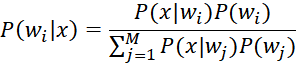其中，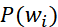是先验概率，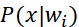是条件概率，我们要求的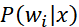是后验概率。

由于分母项在不管求样本的哪个后验概率时都是一样的，实际上我们需要关注的只是分子，因此有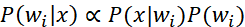2、基于最小错误率的贝叶斯分类理论

接下来阐释基于最小错误率的思想，以及贝叶斯公式在其中如何发挥作用。

假设现在有两类模式，w1和w2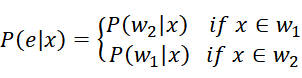就是当x属于w1时却分到了w2的概率，当x属于w2时却分到了w1的概率，这两种概率构成了错误概率

画个样本空间X的后验概率图：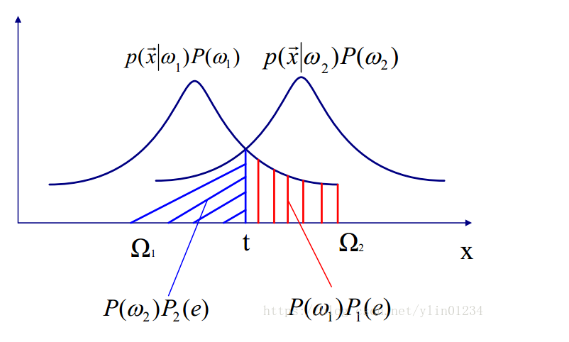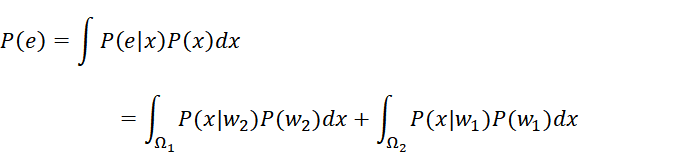在模式分类时，我们的目标是尽量减少分类的错误，即追求最小的错误率，用式子表达就是：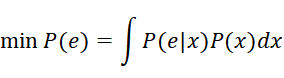这个式子的意思就是对所有的样本x，我们要最小化他们的P(e|x)。

这样看其实还不知道怎么算，但如果使用贝叶斯公式来表达的话就很清楚了。

可以看出最小化错误率就是最大化后验概率，因此在决策的时候我们只需要比较后验概率P(w|x)的大小，

如果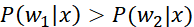则判别为w1，反之则判别为w2。这样我们就能最小化错误率了。

而后验概率可以用贝叶斯公式计算，因此使用贝叶斯公式对样本进行分类的分类器为最小错误率贝叶斯分类器，它的判别函数为：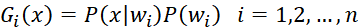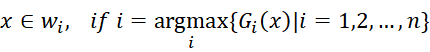上面即为最小错误率贝叶斯分类器。

要计算判别函数值（后验概率），就要先知道先验概率和条件概率。

1）先验概率可以通过计算各类样本在总样本数占的比例来得到，如w1类样本数为5，总样本数为11，那么P(w1)=5/11

2）条件概率使用高斯分布计算：

若样本x为d维向量，第i类wi样本的条件概率密度函数服从均值为mi，协方差为Si的多元高斯分布，条件概率函数为：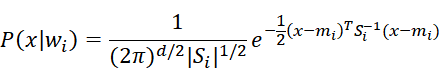3）取对数，最终的判别函数为：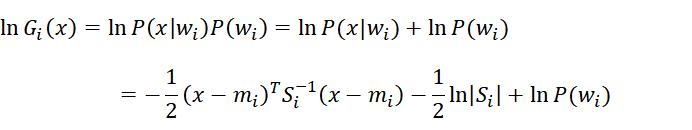（推导的时候有一项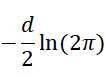为常数项可以省略）

推到这里已经知道我们要做什么了，就是算样本的平均值，协方差，以及先验概率

4）算出样本x的每类Gi(x)值后，取最大的Gi(x)值对应的i，即样本x属于wi类

3、matlab实现

这里使用了教材《模式识别与人工智能（基于matlab）》的一段代码

clear;
clc;
%% 加载样本dataset，包含训练数据和测试数据,数据shape为[样本数，特征维数] %%
train_data = [A_train;B_train;C_train;D_train];
test_data = [A_test;B_test;C_test;D_test];
N1_train = size(A_train, 1); N2_train = size(B_train, 1); N3_train = size(C_train, 1); N4_train = size(D_train, 1); % 各个类别的训练样本数
N_train = N1_train + N2_train + N3_train + N4_train; % 训练样本总数
N1_test = size(A_test, 1); N2_test = size(B_test, 1); N3_test = size(C_test, 1); N4_test = size(D_test, 1); % 各个类别的测试样本的数量
N_test = N1_test + N2_test + N3_test + N4_test; % 测试样本总数
w = 4; % 类别数
n = 3; % 特征数

%% 初始样本数据计算 %%
% 求样本均值
X1 = mean(A_train)'; X2 = mean(B_train)'; X3 = mean(C_train)'; X4 = mean(D_train)';
% 求样本协方差矩阵
S1 = cov(A_train); S2 = cov(B_train); S3 = cov(C_train); S4 = cov(D_train);
% 求协方差矩阵的逆矩阵
S1_ = inv(S1); S2_ = inv(S2); S3_ = inv(S3); S4_ = inv(S4);
% 求协方差矩阵的行列式
S11 = det(S1); S22 = det(S2); S33 = det(S3); S44 = det(S4);
% 先验概率
Pw1 = N1_train/N_train; Pw2 = N2_train/N_train; Pw3 = N3_train/N_train; Pw4 = N4_train/N_train;

%% 计算测试样本的后验概率 %%
for k = 1 : N_test
P1 = -1/2*(test_data(k,:)'-X1)'*S1_*(test_data(k,:)'-X1)-1/2*log(S11)+log(Pw1);
P2 = -1/2*(test_data(k,:)'-X2)'*S2_*(test_data(k,:)'-X2)-1/2*log(S22)+log(Pw2);
P3 = -1/2*(test_data(k,:)'-X3)'*S3_*(test_data(k,:)'-X3)-1/2*log(S33)+log(Pw3);
P4 = -1/2*(test_data(k,:)'-X4)'*S4_*(test_data(k,:)'-X4)-1/2*log(S44)+log(Pw4);
P = [P1 P2 P3 P4];
Pmax = max(P); % 取后验概率最大的那一类
if Pmax == P1
w = 1;
plot3(test_data(k,1), test_data(k,2), test_data(k,3),'ro');
grid on;hold on;
elseif Pmax == P2
w = 2;
plot3(test_data(k,1), test_data(k,2), test_data(k,3),'b>');
grid on;hold on;
elseif Pmax == P3
w = 3;
plot3(test_data(k,1), test_data(k,2), test_data(k,3),'g+');
grid on;hold on;
elseif Pmax == P4
w = 4;
plot3(test_data(k,1), test_data(k,2), test_data(k,3),'y*');
grid on;hold on;
else
return
end
end

运行结果：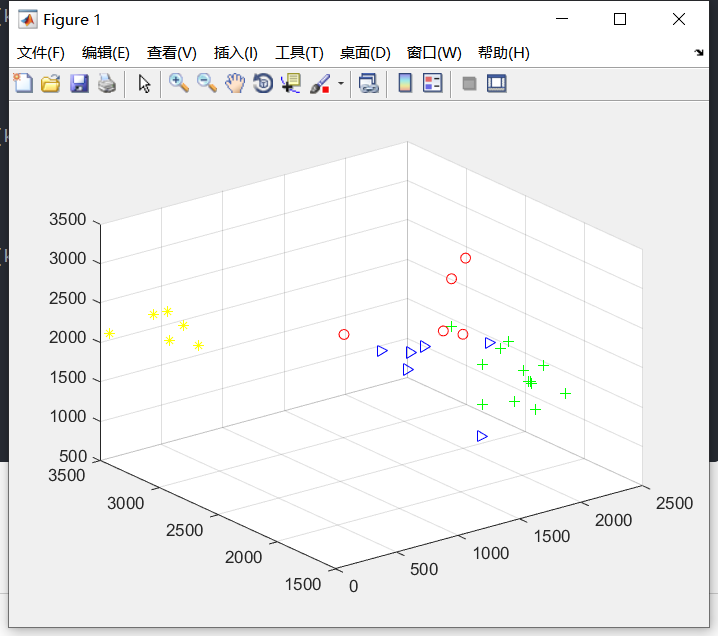分类的结果还是比较好的

欢迎继续学习下一篇【最小风险贝叶斯分类和matlab实现】

展开全文• 三次作业，分别用最小错误率贝叶斯分类器，Fisher线性分类器，人工神经网络进行识别。都采用matlab编程，前两种可在平台上手写数字并识别，人工神经网络版本的没有手写平台，能够通过程序读取图片并返回识别出的数字...
• 1.先验概率：在缺乏某种事实的情况下描述一个变量。比如预测下一次捞到的鱼的类别是很困难的，假设下一次捞到鲈鱼的概率为,捞到鲑鱼的概率为，这里的就是先验...可以通过先验概率计算出，也就是贝叶斯公式： 3...
•贝叶斯分类器
•matlab
• 其中女生类别标号为0，男生类别标号为1，数据集为dataset1.txt，dataset2.txt，dataset3.txt，第一列为身高，第二列为体重，第三列为类别标号分类 贝叶斯
• 1. 最小错误贝叶斯分类器原理 ...可以看出使错误率最小的决策就是使后验概率最大的决策，因此最小错误率贝叶斯决策可写为： 其中，后验概率的计算使用贝叶斯公式计算，因此要已知先验概率p(ωi)以及类条件概率密度p(x算法 分类
• 计算男女身高,非常强大的matlab编程实现贝叶斯程序，可直接使用，模式识别
• 最小错误率贝叶斯分类实验和可运行源代码。可以让读者迅速掌握贝叶斯分类的原理。源代码可编译可运行。贝叶斯分类
• 最小错误率贝叶斯决策和最小风险贝叶斯决策matlab代码，贝叶斯决策理论方法，是统计模式识别中的一个基本方法。贝叶斯决策判据，既考虑了各类参考总体出现的概率大小，又考虑了因误判造成的损失大小，判别能力强。
• 利用MATLAB实现最小错误率贝叶斯判别摘要：matlab软件平台为用户提供了强大的科学计算与可视化功能，具有简单、易用的用户环境，尤其适合矩阵数据的计算处理。根据matlab的特点，将其与模式识别原理结合起来，以油...
• 1. 朴素贝叶斯分类 1.1 理论： 1.2 朴素贝叶斯算法流程： 1.3 举例说明 1.3.1 计算步骤： 1.3.2 程序代码： 2. 贝叶斯估计 2.1 算法流程： 2.2 举例说明 1. 朴素贝叶斯分类 朴素贝叶斯是基于贝叶斯定理与...朴素贝叶斯算法 机器学习 python 算法 分类算法
• 自己编写的，很实用的基于最小错误率贝叶斯决策！
• 含有三份代码，都是用matlab实现，分别是基于贝叶斯，基于朴素贝叶斯，基于最小错误率贝叶斯的手写数字识别代码。其中朴素贝叶斯用到了PCA，准确率达到95%
• 模式识别：最小错误率贝叶斯决策分类

万次阅读 多人点赞 2018-10-13 01:11:24
一、引言 1.用贝叶斯决策理论分类要事先知道两个条件及要求： ①.... 及特征向量的条件概率密度： ...②然后用估计的概率密度设计贝叶斯分类器 前提：训练样本的分布能代表样本的真实分布。每个样本集中...模式识别 贝叶斯决策分类 python
• 模式识别实例：包括最小错误率、最小风险贝叶斯决策matlab代码，注释清楚丰富。
• 两个贝叶斯分类器的例子，分别基于最小错误率与最小风险贝叶斯分类器
• 运用贝叶斯最小错误率法则识别手写数字，直接运行即可手写数字，然后识别。
• 对三维空间特征的数据，通过最小错误率贝叶斯判别方式，对两类数据进行分类，并在空间中绘制出贝叶斯决策面
• 实验原理 利用贝叶斯后验概率确定分类： 实验内容 实验代码模式识别 聚类算法 算法
• 也就是说，贝叶斯分类器是最小错误率意义上的优化，它遵循“多数占优”这一基本原则。 贝叶斯公式 其中，是先验概率，是条件概率，我们要求的是后验概率。 由于分母项在不管求样本的哪个后验概率时都是一样的，...机器学习 模式识别...